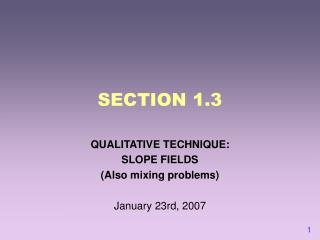DownloadDownload PresentationSECTION 1.3

# SECTION 1.3

Télécharger la présentation## SECTION 1.3

- - - - - - - - - - - - - - - - - - - - - - - - - - - E N D - - - - - - - - - - - - - - - - - - - - - - - - - - -
##### Presentation Transcript

1. SECTION 1.3 QUALITATIVE TECHNIQUE: SLOPE FIELDS (Also mixing problems) January 23rd, 2007

2. Can the computer solve DE? Yes! Weeeell, No. Not always. Many DE’s don’t have “analytic” solutions (i.e. you can’t always find an expression of y in terms of t. Maple can find analytic solutions for DE’s sometimes. Do the Maple handout. Exercise (Maple): Solve Find a solution with ICs T(2) = 100. Find a solution with ICs T(2) = 20.

3. Focus on geometry Finding an exact solution of a DE (i.e. what we did for separable DE’s) isn’t always possible. In fact, it isn’t even possible most of the time! In this section, we’ll study the slope field, an important qualitative tool for analyzing DE’s. It relies on geometry to represent the families of solutions to a DE. This tool is called qualitative because it will not give you a precise formula, but it will give you meaningful (and usually quite accurate) information about the solutions to a DE. In fact, even if we know the exact solution to a DE, we will often do qualitative analysis as well.

4. From last time… Separable Diffy-Q’s Example: Let’s look at some solutions!

5. The slope field You may have noticed that the solutions to the DE kind of “parallel each other.” That is, solutions that are close to each other also have similar slopes. The slope field represents these slopes (dy/dt) on the ty-plane. For each point (y, t) we’ll compute dy/dt by plugging the values of y and t into the original DE. Example: If y = 3, t = 2, and dy/dt = t - y, then at the point (2, 3), we have dy/dt = 2-3 = -1. So we draw a teeny line with slope -1 to indicate that any solution passing through (2, 3) has slope -1 at that point.

6. Using the HPGSolver We are going to plot the slope field for dy/dt = t - y. • Open the Differential Equations software and go to HPGSolver. • Enter t - y as the value for dy/dt and click on Show Field. • Click anywhere on the slope field and it will draw the solution curve through that point. • Now plot the solution for the IVP y(0) = 3 by entering 0 and 3 in the boxes for t0 and y0 and clicking Solution.

7. Now try this… Plot the slope field for and plot the solution y(2) = 1.

8. Some special slope fields Tell me about it: • What should the slope field for dy/dt = f(t) look like? (In this situation, dy/dt depends only on t.) Make a hypothesis and try a few examples on HPGSolver. • What should the slope field for dy/dt = f(y) look like? (In this situation, dy/dt depends only on y.) Make a hypothesis and try a few examples on HPGSolver. These equations, called autonomous, are especially important.

9. Mixology 101 Look at problem 33 on p. 34. • What are the quantities in this problem? s = amount of salt (in pounds) in the water at time t t = time in minutes • What does ds/dt represent (in words)? • How much salt enters the bucket per minute? • How much salt leaves the bucket per minute? • Write a differential equation of the form ds/dt = f(s,t) for this problem. ds/dt = (rate salt is entering) - (rate salt is leaving) ds/dt = 0.25 - (0.1)s • What are the initial conditions for this problem? • Use HPGSolver to plot a slope field and solutions.

10. Partial solution ds/dt = .25 - 0.1s (note that s = 2.5 is the equilibrium) ds/(.25 - 0.1s) = dt integrate both sides ln(|.25 - 0.1s|)/(-.1) = t + c s(t) = ke^(-.1t) + .25/.1 (note that s -> 2.5 when t -> infinity) Question: why does it make sense that s=2.5 is the equilibrium? Answer: equilibrium means “rate of salt coming in = rate of salt going out” Now, rate of salt coming in = .25 lb per min = rate of salt going out = one-tenth of salt, which happens when salt = 2.5 lbs

11. The not-so-chilly chili Read problem 38, p. 35 • What are the quantities in this problem? • Which of these are changing? Express these as derivatives. • What are the initial conditions? • Explain what kind of solutions we should expect. • This problem will be due for homework next Monday.

12. Intro to Lab 1.3 (p. 147) You will do this lab in pairs, and each pair gets one set of parameters from Table 1.10. What techniques can you imagine using in this lab? What homework problem does this remind you of?